Next: 5.4 Implementation Details Up: 5.3 Generation of Random Previous: 5.3.4 Cosine Distribution

## 5.3.5 Direction Vector Sampling Benchmarks

All the algorithms, presented in the previous sections, were implemented using C++ and tested on an Intel Core 2 Duo E6600 processor clocking at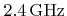. To compare the algorithms for different parameters,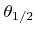was varied, which is the angle at half maximum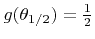. For the power cosine distribution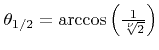and for the coned cosine distribution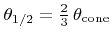.

The runtimes for sampling 100 million random vectors are compared for different algorithms in Table 5.3. Forthe random direction vectors follow a simple cosine distribution for all presented algorithms. For this specific case Algorithm 5.6 is able to compete with Algorithm 5.2 for the power cosine distribution with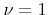and is therefore, due to its simplicity, a serious alternative. Forthe compiler is able to use the square root function instead of the power function, which explains the better runtime. For all other cases, the runtimes for sampling the power cosine distribution are comparable with those for sampling the coned cosine distribution.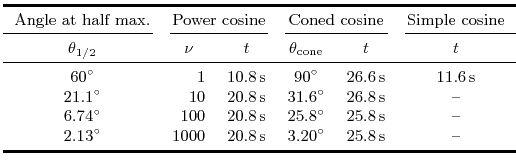Next: 5.4 Implementation Details Up: 5.3 Generation of Random Previous: 5.3.4 Cosine Distribution

Otmar Ertl: Numerical Methods for Topography Simulation